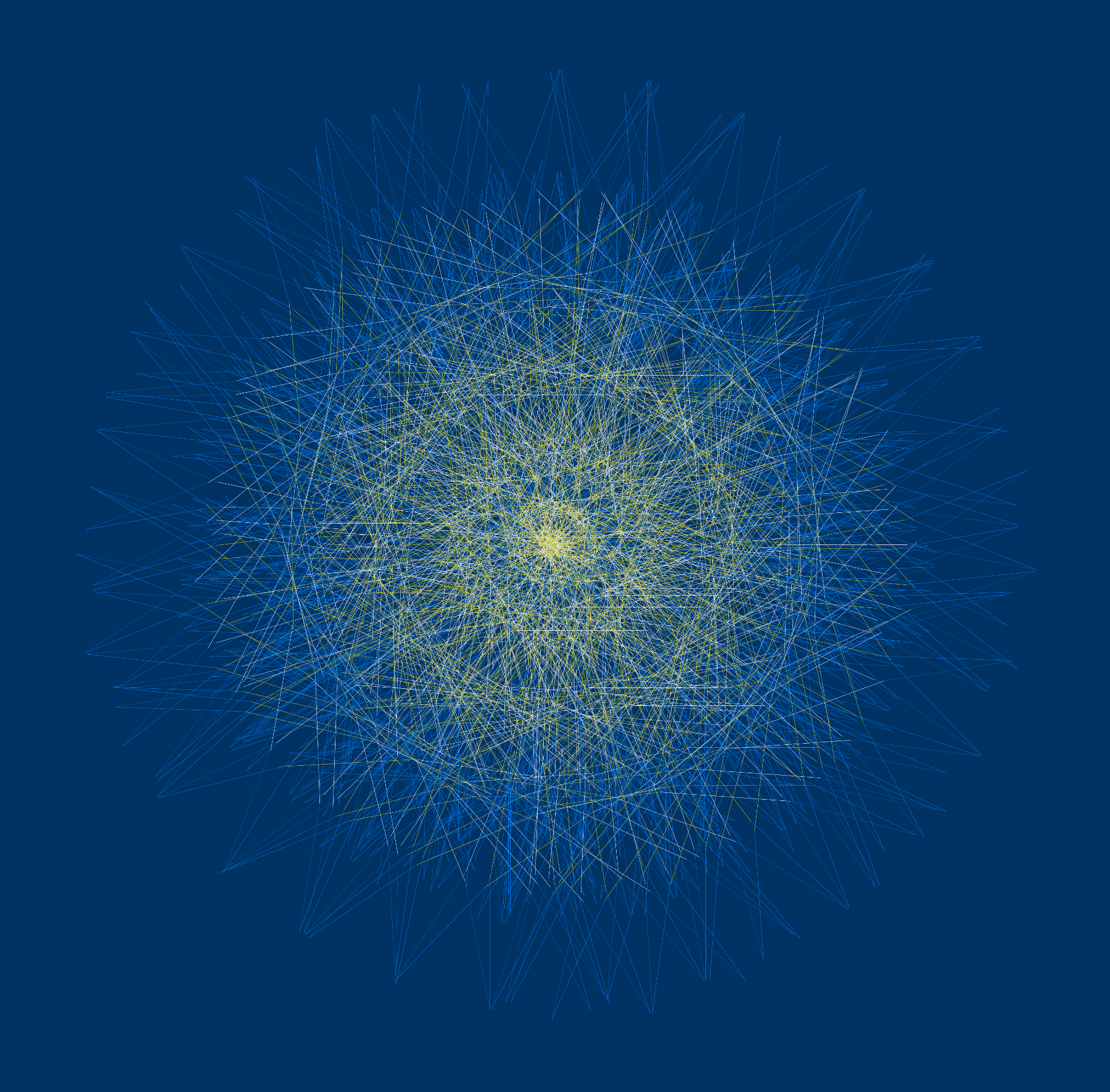# Vincent J. Matsko

Assistant Professor
Department of Mathematics and Statistics, University of San Francisco
San Francisco, CA, USA

Computer-generated art involves ideas not conceivable before the advent of modern technology. My recent work involves randomness on a large scale, experimentation with color, and integrating thousands, sometime millions, of individual elements in a single composition.

Work in this exhibition explores creating images consisting of line segments. Two of the pieces involve varying the angles in the well-known recursive algorithm for generating the Koch snowflake, while the third adapts an algorithm for creating a spiral as an envelope of lines.Fractal Curve +11 +191
20 x 20 cm
Digital print
2015

The Koch snowflake is a fractal represented by the string “F +60 F -120 F +60 F,” where “F” means move forward by a specified length, “+60” means turn counterclockwise 60 degrees, and “-120” means turn clockwise 120 degrees. Each occurrence of “F” is recursively replaced with a copy of the string, which is repeated as many levels as desired. When these instructions are carried out graphically, the Koch snowflake is produced. In this piece, the same algorithm is used, but with different angles. The title of the piece is taken from the particular choice of angles used to create it. The fractal nature of each curve varies, but as the original algorithm derives from producing a fractal, a liberty is taken in calling this a “fractal image."Spiral 1
20 x 20 cm
Digital print
2016

A typical way to create a spiral as a mathematical envelope involves beginning with two points on a circle spaced 180 degrees apart. The points then move around the circle, but at different rates - so that as segments connecting the points are drawn, they become smaller and smaller until the points coincide. By varying the initial separation of the points and the rates at which the points move, as well as allowing the points to move on different circles, a wide variety of images may be produced. In this example, the initial points are 75 degrees apart, the rates at which the points move are in the ratio 9 : 8, and the points move on circles with radii in the ratio 5 : 4.